## The specific heat capacities of some metals (in J g−1 K−1) are given below. Copper: 0.385 Magnesium: 1.020 Mercury: 0.138 Platinum: 0.130 If

Question

The specific heat capacities of some metals (in J g−1 K−1) are given below. Copper: 0.385 Magnesium: 1.020 Mercury: 0.138 Platinum: 0.130 If 100 kJ of heat is added to 10.0 g samples of each of the metals above, all at 25°C, determine which metal will have the smallest increase in temperature.

in progress 0
5 months 2021-08-22T05:46:25+00:00 1 Answers 60 views 0

## Answers ( )

1. Answer: Magnesium metal will have the smallest increase in temperature.

Explanation:

Given: Specific heats are as follows.

Copper = 0.385 J/g K,     Magnesium = 1.020 J/g K

Mercury = 0.138 J/g K,     Platinum = 0.130 J/g K

Heat energy = 100 kJ  (1 kJ = 1000 J) = 100000 J

mass = 10.0 g

Initial temperature =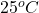Formula used to calculate the increase in temperature for each of the given elements is as follows.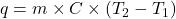where,

q = heat energy

m = mass

C = specific heat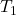= initial temperature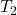= final temperature

• The change in temperature for copper is calculated as follows.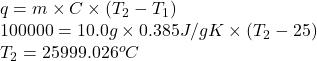• The change in temperature for magnesium is calculated as follows.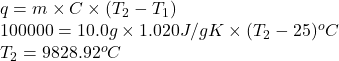• The change in temperature for mercury is calculated as follows.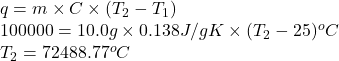• The change in temperature for platinum is calculated as follows.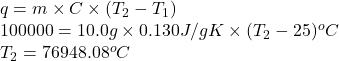Thus, we can conclude that magnesium metal will have the smallest increase in temperature.